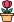엔지니어 게시판
LeetCode 솔루션 분류

# [10/2] 1155. Number of Dice Rolls With Target Sum

### 작성자 정보

•작성
• 작성일

• 1,088 조회
• 0 추천
• 0 비추천

### 본문

Medium

You have `n` dice and each die has `k` faces numbered from `1` to `k`.

Given three integers `n``k`, and `target`, return the number of possible ways (out of the `kn` total ways) to roll the dice so the sum of the face-up numbers equals `target`. Since the answer may be too large, return it modulo `109 + 7`.

Example 1:

```Input: n = 1, k = 6, target = 3
Output: 1
Explanation: You throw one die with 6 faces.
There is only one way to get a sum of 3.
```

Example 2:

```Input: n = 2, k = 6, target = 7
Output: 6
Explanation: You throw two dice, each with 6 faces.
There are 6 ways to get a sum of 7: 1+6, 2+5, 3+4, 4+3, 5+2, 6+1.
```

Example 3:

```Input: n = 30, k = 30, target = 500
Output: 222616187
Explanation: The answer must be returned modulo 109 + 7.
```

Constraints:

• `1 <= n, k <= 30`
• `1 <= target <= 1000`
Accepted
145,563
Submissions
281,436

댓글 0
등록된 댓글이 없습니다.
전체 380 / 1 페이지

• 등록일 11:10
• 등록일 09.28
• 등록일 09.27
• 등록일 09.27
• 등록일 09.27
• 등록일 09.27
• 등록일 09.26
• 등록일 09.26

• 등록일 08.22
• 등록일 09.07
• 등록일 09.07
• 등록일 09.08
• 등록일 09.08
• 등록일 09.08
• 등록일 09.08
• 등록일 09.09

• 등록일 09.27
• 등록일 09.26
• 등록일 09.26
• 등록일 09.23
• 등록일 09.22

### Stats

• 현재 접속자 240 명
• 오늘 방문자 4,647 명
• 어제 방문자 5,872 명
• 최대 방문자 6,163 명
• 전체 회원수 768 명